0
2013
Impact Factor

# The Possibility of Introducing of Metric Structure in Vortex Hydrodynamic Systems

2018, Vol. 14, no. 4, pp.  495-501

Author(s): Fimin N. N., Chechetkin V. M.

Geometrization of the description of vortex hydrodynamic systems can be made on the basis of the introduction of the Monge – Clebsch potentials, which leads to the Hamiltonian form of the original Euler equations. For this, we construct the kinetic Lagrange potential with the help of the flow velocity field, which is preliminarily determined through a set of scalar Monge potentials, and thermodynamic relations. The next step is to transform the resulting Lagrangian by means of the Legendre transformation to the Hamiltonian function and correctly introduce the generalized impulses canonically conjugate to the configuration variables in the new phase space of the dynamical system. Next, using the Hamiltonian function obtained, we define the Hamiltonian space on the cotangent bundle over the Monge potential manifold. Calculating the Hessian of the Hamiltonian, we obtain the coefficients of the fundamental tensor of the Hamiltonian space defining its metric. Next, we determine analogs of the Christoffel coefficients for the N-linear connection. Considering the Euler – Lagrange equations with the connectivity coefficients obtained, we arrive at the geodesic equations in the form of horizontal and vertical paths in the Hamiltonian space. In the case under study, nontrivial solutions can have only differential equations for vertical paths. Analyzing the resulting system of equations of geodesic motion from the point of view of the stability of solutions, it is possible to obtain important physical conclusions regarding the initial hydrodynamic system. To do this, we investigate a possible increase or decrease in the infinitesimal distance between the geodesic vertical paths (solutions of the corresponding system of Jacobi – Cartan equations). As a result, we can formulate very general criterions for the decay and collapse of a vortex continual system.
Keywords: vortex dynamics, geodesic deviation, Monge manifold, metric tensor
Citation: Fimin N. N., Chechetkin V. M., The Possibility of Introducing of Metric Structure in Vortex Hydrodynamic Systems, Rus. J. Nonlin. Dyn., 2018, Vol. 14, no. 4, pp.  495-501
DOI:10.20537/nd180405

## References

 Arnold, V. I., “Sur la géométrie différentielle des groupes de Lie de dimension infinie et ses applications à l'hydrodynamique des fluides parfaits”, Ann. Inst. Fourier (Grenoble), 1:1 (1966), 319–361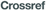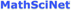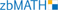Casetti, L., Pettini, M., and Cohen, E. G. D., “Geometric Approach to Hamiltonian Dynamics and Statistical Mechanics”, Phys. Rep., 337:3 (2000), 237–341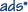Chueshov, I. D., Introduction to the Theory of Infinite-Dimensional Dissipative Systems, ACTA, Kharkiv, 2002, 436 pp.Fimin, N. N., Orlov, Yu. N., and Chechetkin, V. M., “Thermodynamic Properties of Vortex Systems”, Math. Models Comput. Simul., 8:2 (2016), 149–154; Mat. Model., 27:9 (2015), 81–88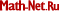Holm, D. D., Marsden, J. E., Ratiu, T., and Weinstein, A., “Nonlinear Stability of Fluid and Plasma Equilibria”, Phys. Rep., 123:1–2 (1985), 116 pp.Kambe, T., “Geometrical Theory of Two-Dimensional Hydrodynamics with Special Reference to a System of Point Vortices”, Fluid Dynam. Res., 33:1–2 (2003), 223–249Kozlov, V. V., Dynamical Systems 10: General Theory of Vortices, Encyclopaedia Math. Sci., 67, Springer, Berlin, 2003, 184 pp.Miron, R., Hrimiuc, D., Shimada, H., and Sabau, S. V., The Geometry of Hamilton and Lagrange Spaces, Kluwer, Dordrecht, 2001, xvi+338 pp.van Saarloos, W., Bedeaux, D., and Mazur, P., “Hydrodynamics for an Ideal Fluid: Hamiltonian Formalism and Liouville Equation”, Phys. A, 107:1 (1981), 109–125Vasylkevych, S. and Marsden, J. E., “The Lie – Poisson Structure of the Euler Equations of an Ideal Fluid”, Dyn. Partial Differ. Equ., 2:4 (2005), 281–300Vedenyapin, V. V. and Fimin, N. N., “The Hamilton – Jacobi Method in a Non-Hamiltonian Situation and Hydrodynamic Substitution”, Dokl. Math., 91:2 (2015), 154–157; Dokl. Akad. Nauk, 461:2 (2015), 136–139 (Russian)